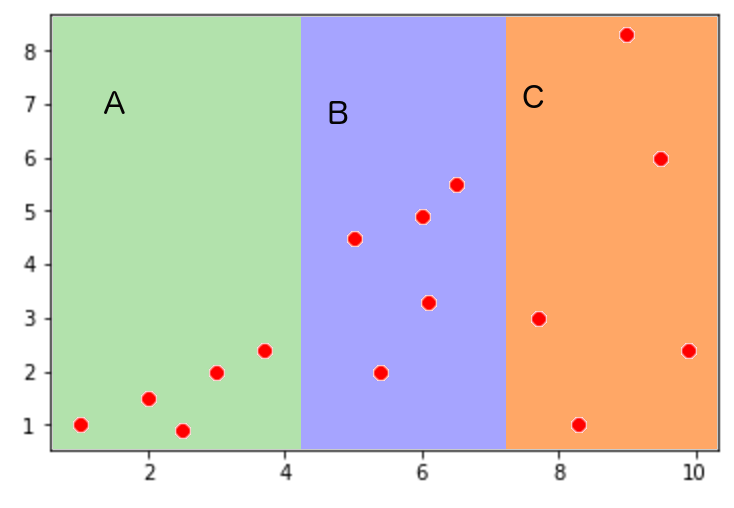### Machine Learning

Linear regression is clearly a very useful tool. Whether you are analyzing crop yields or estimating next year’s GDP, it is always a powerful machine learning technique.

However, it does have limitations. Possibly the most obvious is that it will not be effective on data which isn’t linear. Using linear regression means assuming that the response variable changes linearly with the predictor variables.

# Limitations of Linear Regression

Alfred’s done some thinking, and he wants to account for fertilizer in his tree growing efforts. Assume that for every ton of fertilizer he uses each seed is about 1.5 times more likely to sprout.

Over the past few years, he has compiled a large data set in which he records fertilizer use, seeds planted, and trees sprouted. Is ordinary linear regression likely to give good predictions for the number of sprouting trees given the amount of fertilizer used and number of seeds planted?

# Limitations of Linear Regression

Outliers are another confounding factor when using linear regression. These are elements of a data set that are far removed from the rest of the data.

Outliers are problematic because they are often far enough from the rest of the data that the best-fit line will be strongly skewed by them, even when they are present because of a mistake in recording or an unlikely fluke.

Commonly, outliers are dealt with simply by excluding elements which are too distant from the mean of the data. A slightly more complicated method is to model the data and then exclude whichever elements contribute disproportionately to the error.

It is not impossible for outliers to contain meaningful information though. One should be careful removing test data.

# Limitations of Linear Regression

Another major setback to linear regression is that there may be multicollinearity between predictor variables. This is the term for when several of the input variables appear to be strongly related.

Multicollinearity has a wide range of effects, some of which are outside the scope of this lesson. However, the major concern is that multicollinearity allows many different best-fit equations to appear almost equivalent to a regression algorithm.

As a result, tools such as least squares regression tend to produce unstable results when multicollinearity is involved. There are generally many coefficient values which produce almost equivalent results. This is often problematic, especially if the best-fit equation is intended to extrapolate to future situations where multicollinearity is no longer present.

Another issue is that it becomes difficult to see the impact of single predictor variables on the response variable. For instance, say that two predictor variables $x_1$ and $x_2$ are always exactly equal to each other and therefore perfectly correlated. We can immediately see that multiple weightings, such as $m \cdot x_1 + m\cdot x_2$ and $2m\cdot x_1 + 0\cdot x_2$, will lead to the exact same result. Now it’s impossible to meaningfully predict how much the response variable will change with an increase in $x_1$ because we have no idea which of the possible weightings best fits reality. This both decreases the utility of our results and makes it more likely that our best-fit line won’t fit future situations.

# Limitations of Linear Regression

We can see the effects of multicollinearity clearly when we take the problem to its extreme. Say that we have two predictor variables, $x_1$ and $x_2$, and one response variable $y$. Using the test data given in the table below, determine which candidate best-fit equation has the lowest SSE: $\begin{array}{c|c|c} x_1 & x_2 & y \\ \hline 5&10&3 \\ \hline 2 & 4 & 1\\ \hline 7 & 14 & 6 \\ \hline 2.5 & 5 & 2 \\ \end{array}$

# Limitations of Linear Regression

The property of heteroscedasticity has also been known to create issues in linear regression problems. Heteroscedastic data sets have widely different standard deviations in different areas of the data set, which can cause problems when some points end up with a disproportionate amount of weight in regression calculations.

A data set is displayed on the scatterplot below. Which section of the graph will have the greatest weight in linear regression?# Limitations of Linear Regression

Another classic pitfall in linear regression is overfitting, a phenomenon which takes place when there are enough variables in the best-fit equation for it to mold itself to the data points almost exactly.

Although this sounds useful, in practice it means that errors in measurement, outliers, and other deviations in the data have a large effect on the best-fit equation. An overfitted function might perform well on the data used to train it, but it will often do very badly at approximating new data. Useless variables may become overvalued in order to more exactly match data points, and the function may behave unpredictably after leaving the space of the training data set.

×